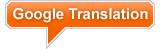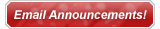Gallery Section### Lens Activity: Looking Through Lenses

A lens is a piece of transparent material with at least one curved surface, which refracts, or bends, light rays coming from an object. Lenses are usually made out of glass or plastic and they have special characteristics. In this activity that follows you will look at only two kinds of lenses, convex and concave, and observe their similarities and differences. You will also be testing out what happens when you use multiple lenses at the same time.

# Required Materials

• Science notebook

• 2 convex lenses

• 2 concave lenses

• Flashlight

• White paper

# Activity Directions

1. Look closely at the lenses and answer these questions in your science notebook:
• How are the lenses shaped?

• How are the lenses alike?

• How are the lenses different?

2. Look through the lenses at the pages of a book, your hands, a hair, and other things. Draw what you see in your science notebook and label each picture with the type of lens with which you observed the object. Be sure to answer the following questions:
• How does a concave lens make things look?

• How does a convex lens make things look?

3. Lenses bend light in different directions. Shine a flashlight through the lenses onto a piece of white paper and then answer the following questions in your science notebook:
• In what direction do convex lenses bend light?

• In what direction do concave lenses bend light?

4. Shine the flashlight through different combinations of lenses: two convex lenses, two concave lenses, one concave and one convex lens. Draw pictures of what you see in your science notebook and answer these questions:
• What happens when you use multiple lenses at the same time?

• Can you use two different lenses to make things far away appear closer?

5. If you can, darken the room and place a convex lens between a sunlit window and a white piece of paper. Place the lens close to the paper and then slowly move the lens towards the window. Draw a picture of what you see in your science notebook.

Want to Learn More? Read an article related to this activity: